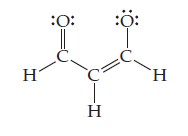# Problem: Consider the Lewis structure shown below.Does the Lewis structure depict a neutral molecule or an ion?

###### FREE Expert Solution

H: FC = 1 – (1 + 0) = 0

left C: FC = 4 – (4+0) = 0

middle C: FC = 4 – (4+0) = 0

right C: FC = 4 – (4+0) = 0

left O: FC = 6 – (2+4) = 0

right O: FC = 6 – (1+6) = -1

96% (42 ratings)###### Problem Details

Consider the Lewis structure shown below.Does the Lewis structure depict a neutral molecule or an ion?

Frequently Asked Questions

What scientific concept do you need to know in order to solve this problem?

Our tutors have indicated that to solve this problem you will need to apply the Formal Charge concept. You can view video lessons to learn Formal Charge. Or if you need more Formal Charge practice, you can also practice Formal Charge practice problems.

What professor is this problem relevant for?

Based on our data, we think this problem is relevant for Professor Williams' class at ASU.